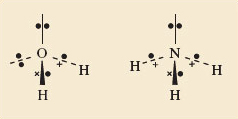Science, Maths & Technology

### Become an OU student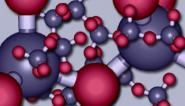The molecular world

Start this free course now. Just create an account and sign in. Enrol and complete the course for a free statement of participation or digital badge if available.

# 6.2 The shapes of some molecules

Here we shall look at the shapes of some simple molecules of the typical elements. In doing so, we shall meet the problem of representing three-dimensional shapes on two-dimensional paper. Let's use methane, CH4, as an example. A ball-and-stick representation of this tetrahedral molecule is shown in Figure 45. To draw such structures in this course, we shall often make use of the 'flying-wedge notation'. A flying-wedge representation of the methane molecule of Figure 45 is shown in Figure 46. The atom at the pointed or thin end of the wedge is assumed to be in the plane of the paper, and the atom at the thick end is in front of the plane. The connection between this wedge and the perspective of Figure 45 is obvious. A continuous line (—) joins two atoms that both lie in the plane of the paper. A dashed line (- -) joins together two atoms, one of which is in the plane of the paper, whereas the other is behind it.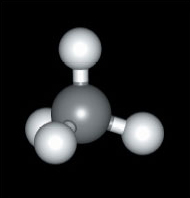Figure 45 A ball-and-stick model of methane, CH4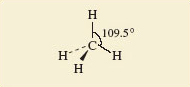Figure 46 A flying-wedge representation of methane

Having established this convention, we shall now examine the shapes of some simple fluorides. In Section 2, we reminded you how to use the Periodic Table to predict the highest fluoride of a typical element.

## Question 36

Use Figure 18 to predict the highest fluorides of beryllium, boron, carbon, iodine, phosphorus and sulfur.

The Group numbers are: beryllium, II; boron, III; carbon, IV; phosphorus, V; sulfur, VI; iodine, VII. The predicted highest fluorides are therefore BeF2, BF3, CF4, PF5, SF6 and IF7.

These predictions are correct. All these molecules exist, and their shapes, which have been experimentally determined, are shown in Figure 47.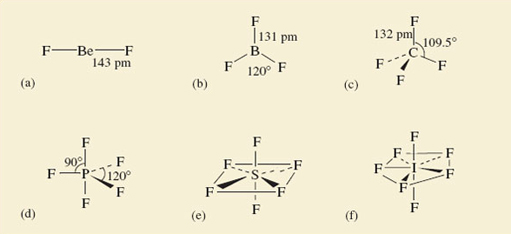Figure 47 The shapes of some fluoride molecules: (a) BeF2; (b) BF3; (c) CF4; (d) PF5; (e) SF6; (f) IF7

Beryllium difluoride is a glassy non-molecular solid at room temperature, but the BeF2 molecule (Figure 47a) is obtained when the solid is vaporized by heating it to 1 200 °C. It is linear; that is, the sequence of atoms F—Be—F lies on a straight line. The spatial arrangement of the neighbouring atoms around a particular atom is said to be the coordination of that atom. In BeF2, therefore, the beryllium is in linear coordination.

At 25 °C, BF3, CF4, PF5, SF6 and IF7 are all gases containing molecules with the shapes shown in Figure 47b-f. In BF3, all four atoms lie in the same plane, the boron atom forming three B—F bonds to three fluorine atoms at the corners of an equilateral triangle (see Maths Help below). This arrangement of fluorines around boron is called trigonal planar. In CF4, we have the tetrahedral coordination around carbon that we have already noted in methane (Figures 45 and 46)

## Maths help: equilateral triangles

An equilateral triangle (Figure 48) has three equal sides, and three internal angles of 60°. The three fluorine atoms in BF3 lie at the corners of an equilateral triangle. The four faces of a regular tetrahedron, like the one whose corners are defined by the four hydrogen atoms of methane (Figure 45), are equilateral triangles. So are the eight faces of a regular octahedron, like the one whose corners are defined by the six chloride ions around each sodium ion in NaCl (Figure 10b).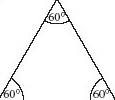Figure 48 An equilateral triangle

The coordination in PF5, SF6 and IF7 is best described by starting with the horizontal planes containing the central atom of these molecules. In PF5, this plane contains three P—F bonds directed towards the corners of an equilateral triangle as in BF3; in SF6, it contains the sulfur atom with four surrounding fluorines at the corners of a square.

## Question 37

What does this horizontal plane contain in IF7?

The iodine atom, and five I—F bonds directed towards five fluorine atoms at the corners of a regular pentagon.

In all three cases, the coordination is then completed by two other bonds to fluorine at 90° to those in the horizontal plane, one pointing up, and the other pointing down. These arrangments in PF5, SF6 and IF7 are called trigonal bipyramidal, octahedral and pentagonal bipyramidal, respectively.

In the octahedral molecule SF6, all the fluorine atoms are equivalent. From each of the fluorine atoms, the view of the rest of the molecule looks the same. But in PF5 and IF7, this is not so. There are two kinds of fluorine position: equatorial positions in the horizontal plane, and axial positions at right-angles to it. In Figure 49, these two kinds of position are labelled for the trigonal-bipyramidal arrangement in PF5.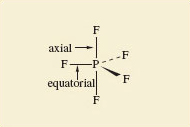Figure 49 Axial and equatorial positions in PF5

Why are such arrangements adopted? We can imagine that SF6might have the shape shown in Structure 7.3, where the sulfur atom has six S﻿—﻿F bonds directed towards the corners of a regular hexagon, and all seven atoms are in the same plane. But experiment shows the actual shape is the octahedral one shown in Figure 47e.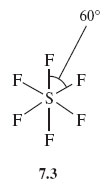## Question 38

Suggest a reason for this preference.

What we are looking for is the idea that the S—F bonds repel one another, so that they get as far apart in space as possible. In Structure 7.3, they are confined to a single plane and the angle between them is only 60°. By adopting octahedral coordination, the greatest possible separation of the S—F bonds is achieved, the angle being increased to 90°.

All the shapes shown in Figure 47 conform to this principle by enforcing a good separation of the bonds in space. The principle looks even more reasonable when we remember that we have identified the bonds with pairs of electrons; the like charges of these electron pairs lead to an expectation that one pair will repel another.

However, two very common molecules will soon dispel the notion that repulsion between bonding pairs of electrons is the sole determinant of molecular shape. These are water, H2O, and ammonia, NH3.

## Question 39

What would be the shapes of H2O and NH3 molecules if they were dictated only by bond-bond repulsions?

The two O—H bonds of H2O and the three N—H bonds of NH3 would get as far apart as possible: H2O would be linear like BeF2, and NH3 would be trigonal planar like BF3 (see Figure 47).

The observed shapes are shown in Structures 7.4 and 7.5. H2O is V-shaped and NH3 is pyramidal. In both cases the inter-bond angle is much closer to the tetrahedral angle of 109.5° than to our predicted values of 180° and 120°, respectively.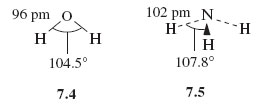## Question 40

What is present in H2O and NH3 that might explain these deviations?